Question

# The cantilevered beam shown here has a known rigidity, EI, andlength, b, and is loaded...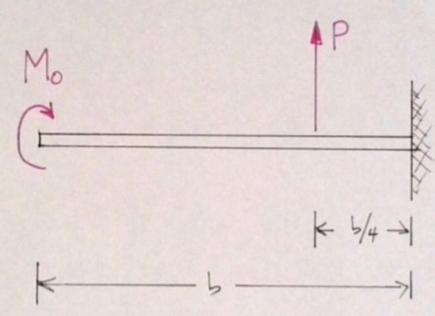The cantilevered beam shown here has a known rigidity, EI, and length, b, and is loaded with a point force and a point moment as shown

a) Determine all reactions forces and draw the shear and moment diagrams for this loading.

b) Using discontinuity functions and the integration method, find the deflection and the slope of the beam at the free end.

c) Using the moment-area method, find the deflection and the slope of the beam at the location of the point load.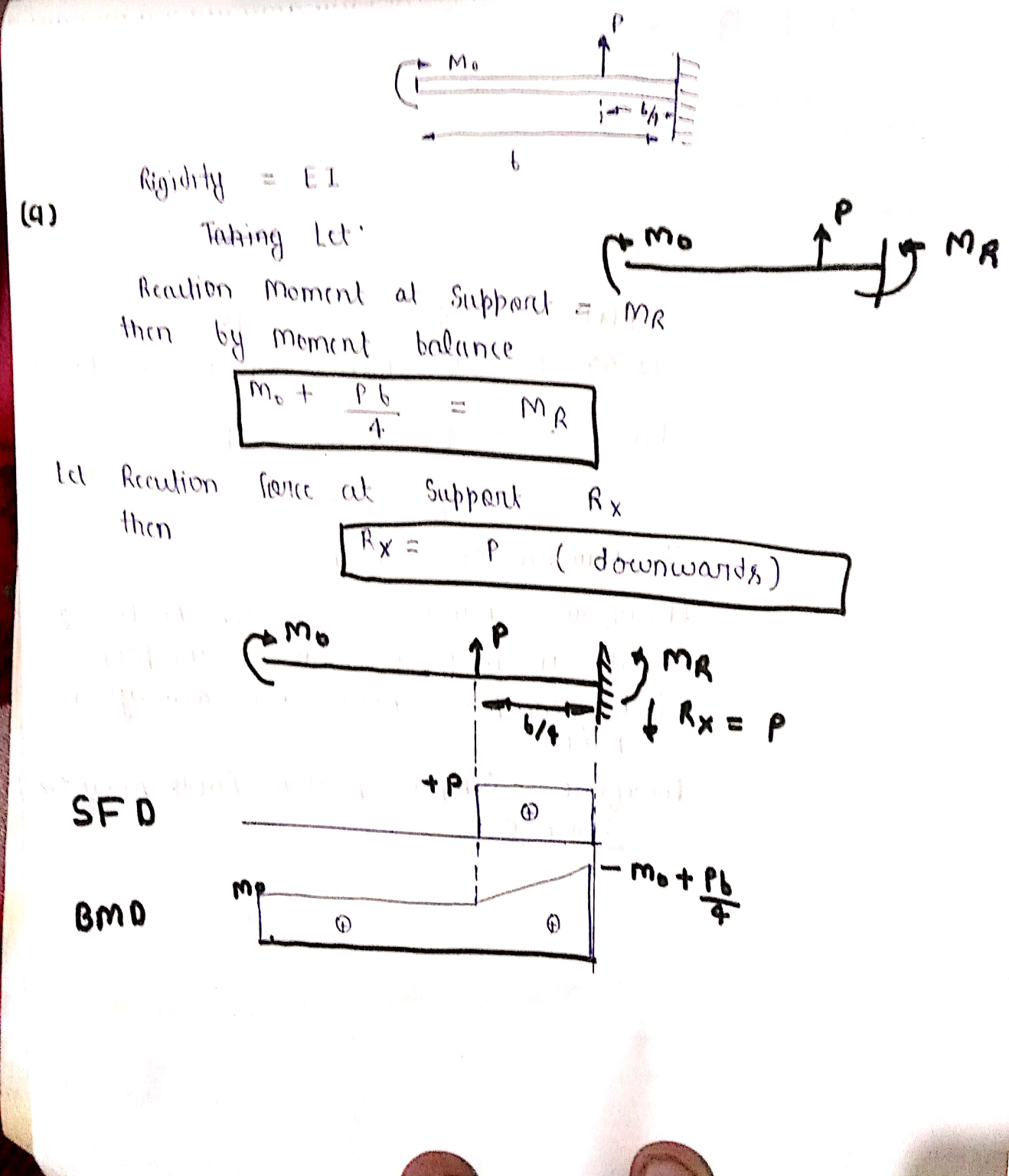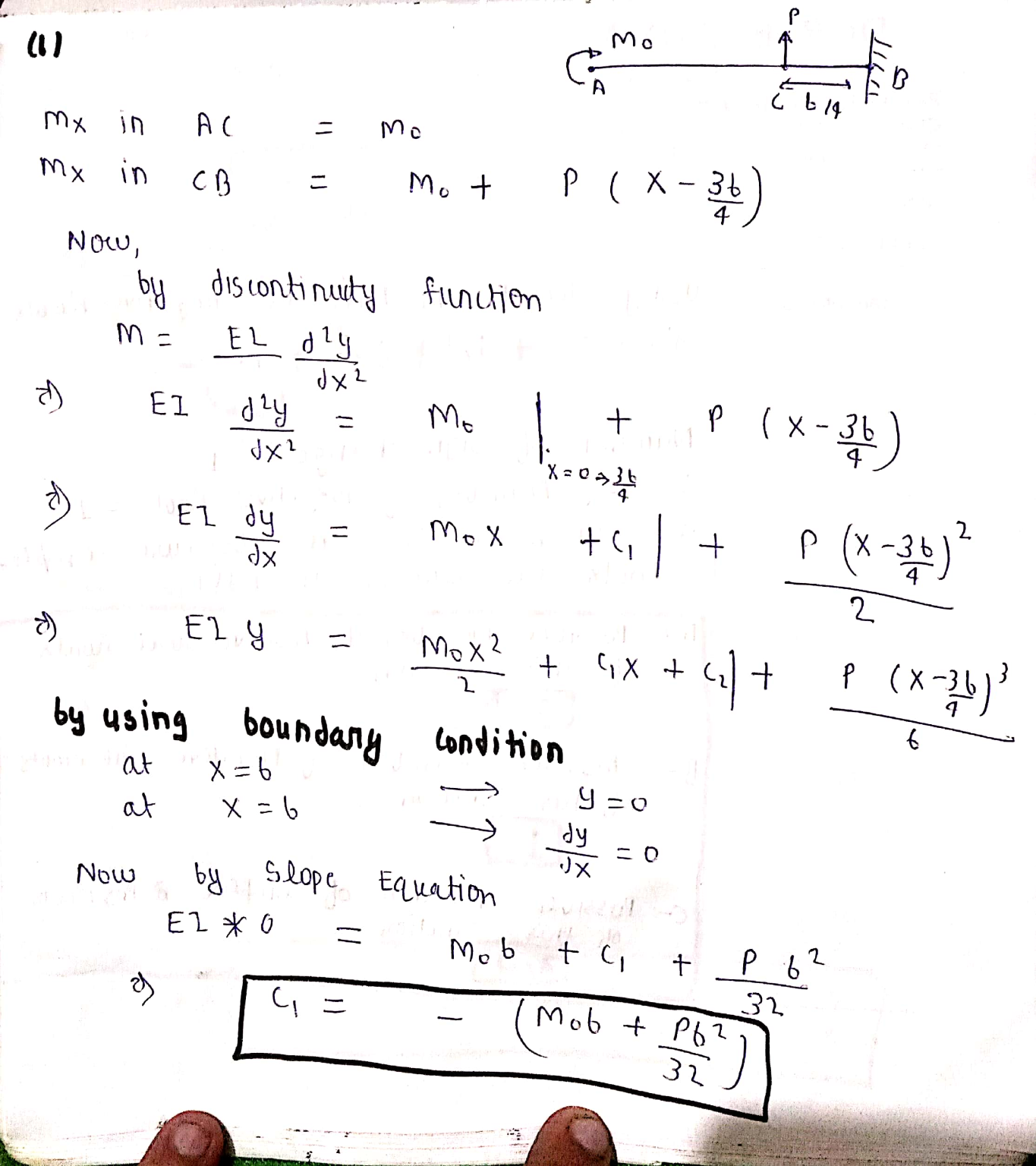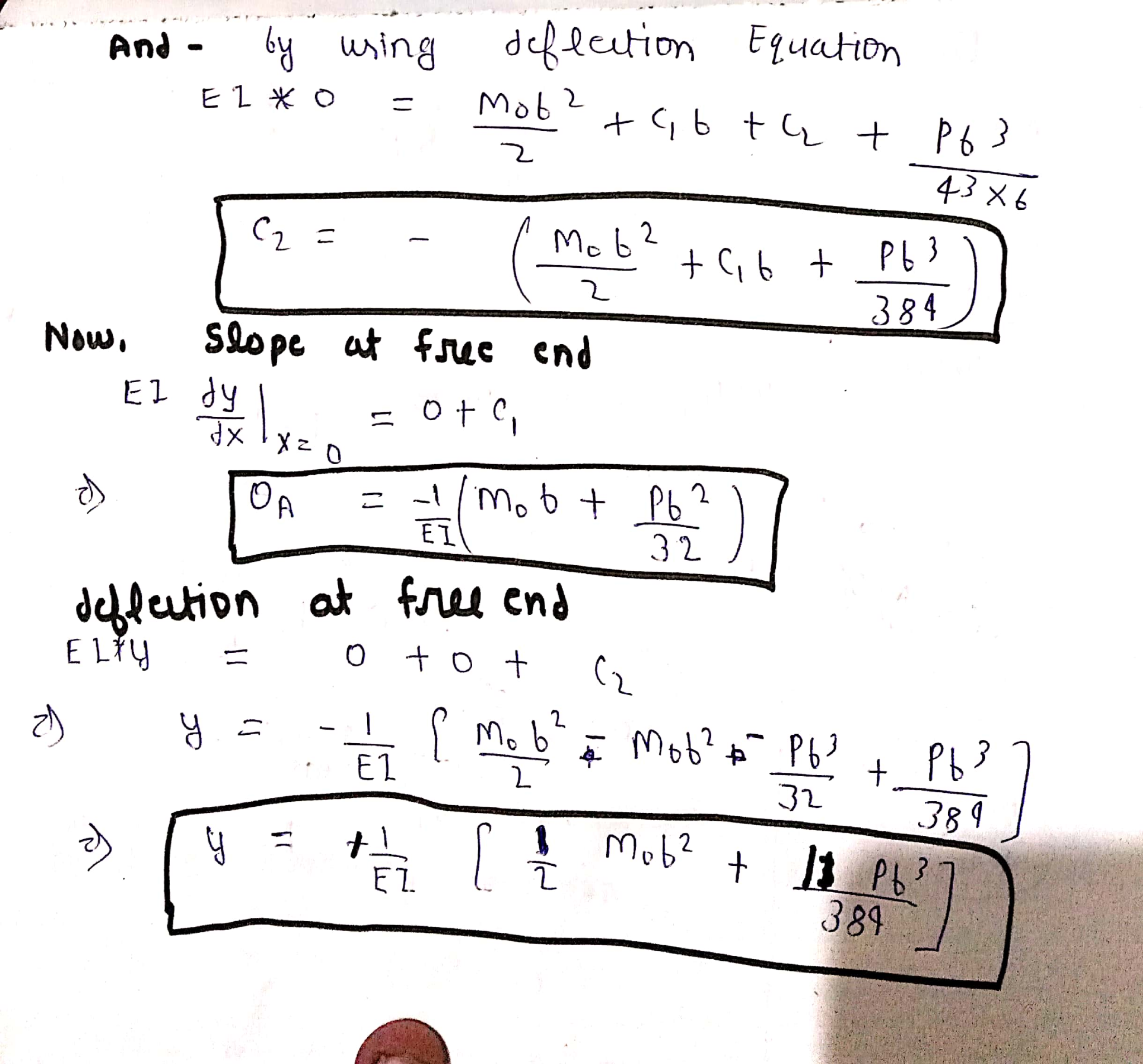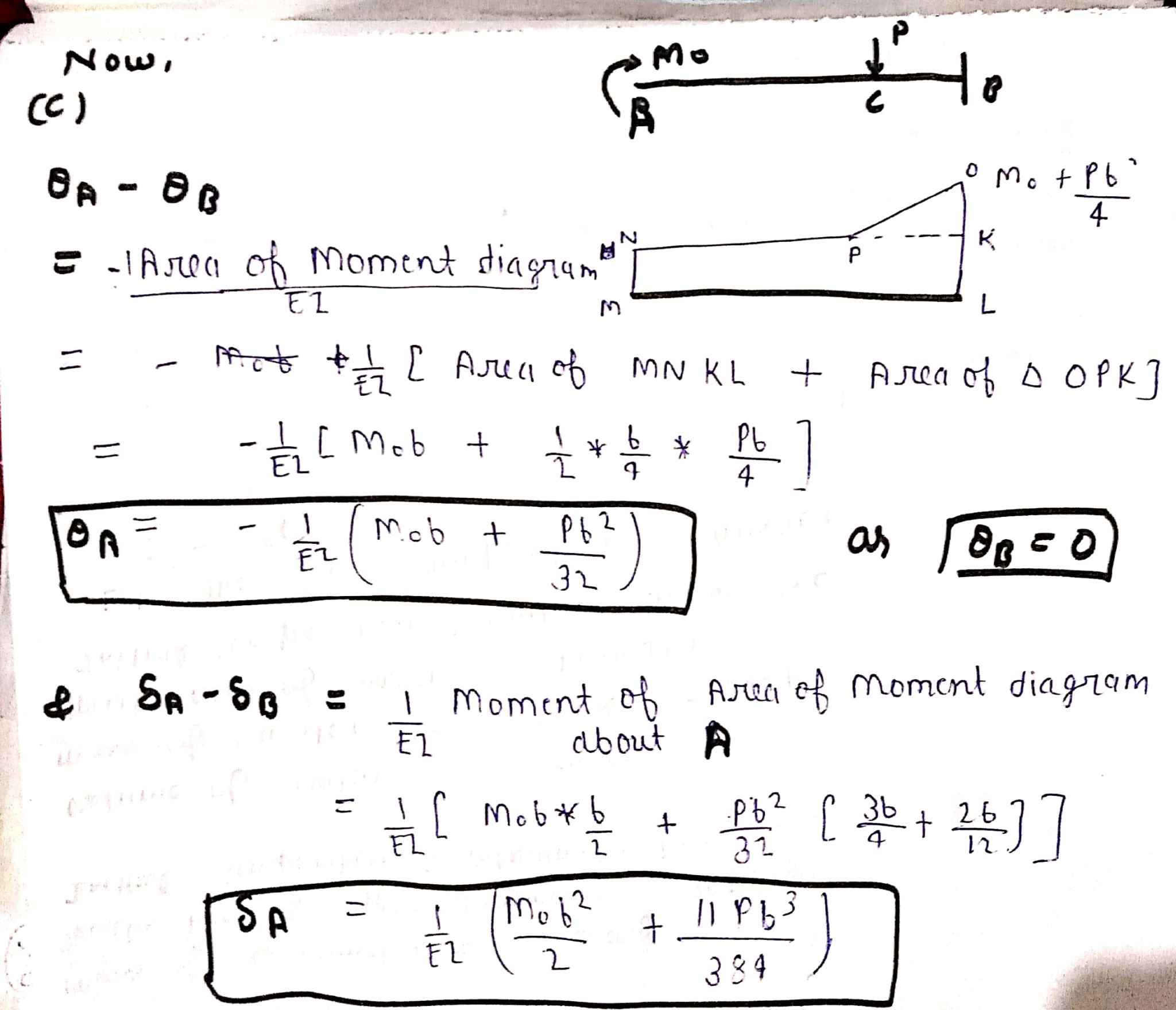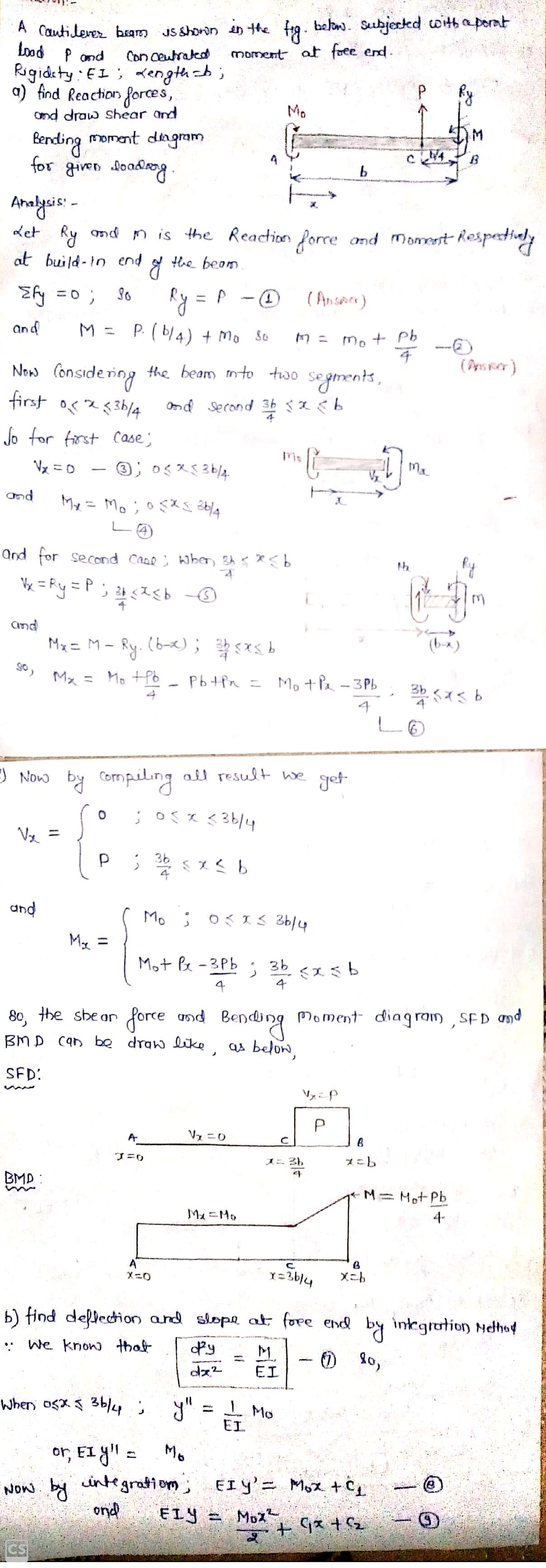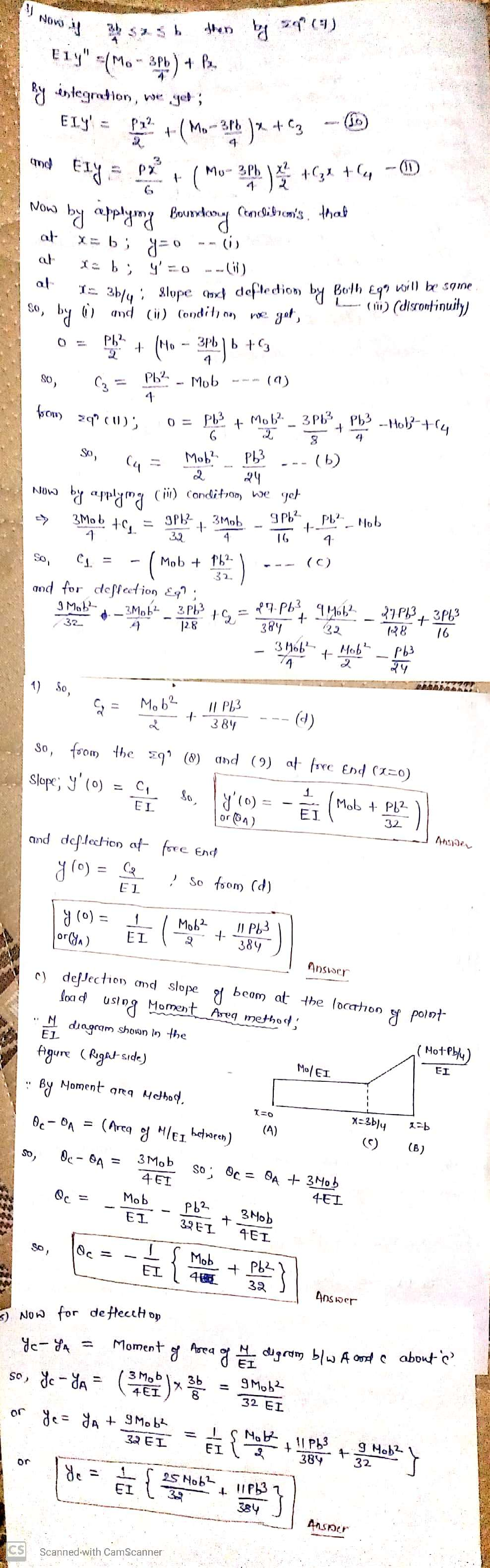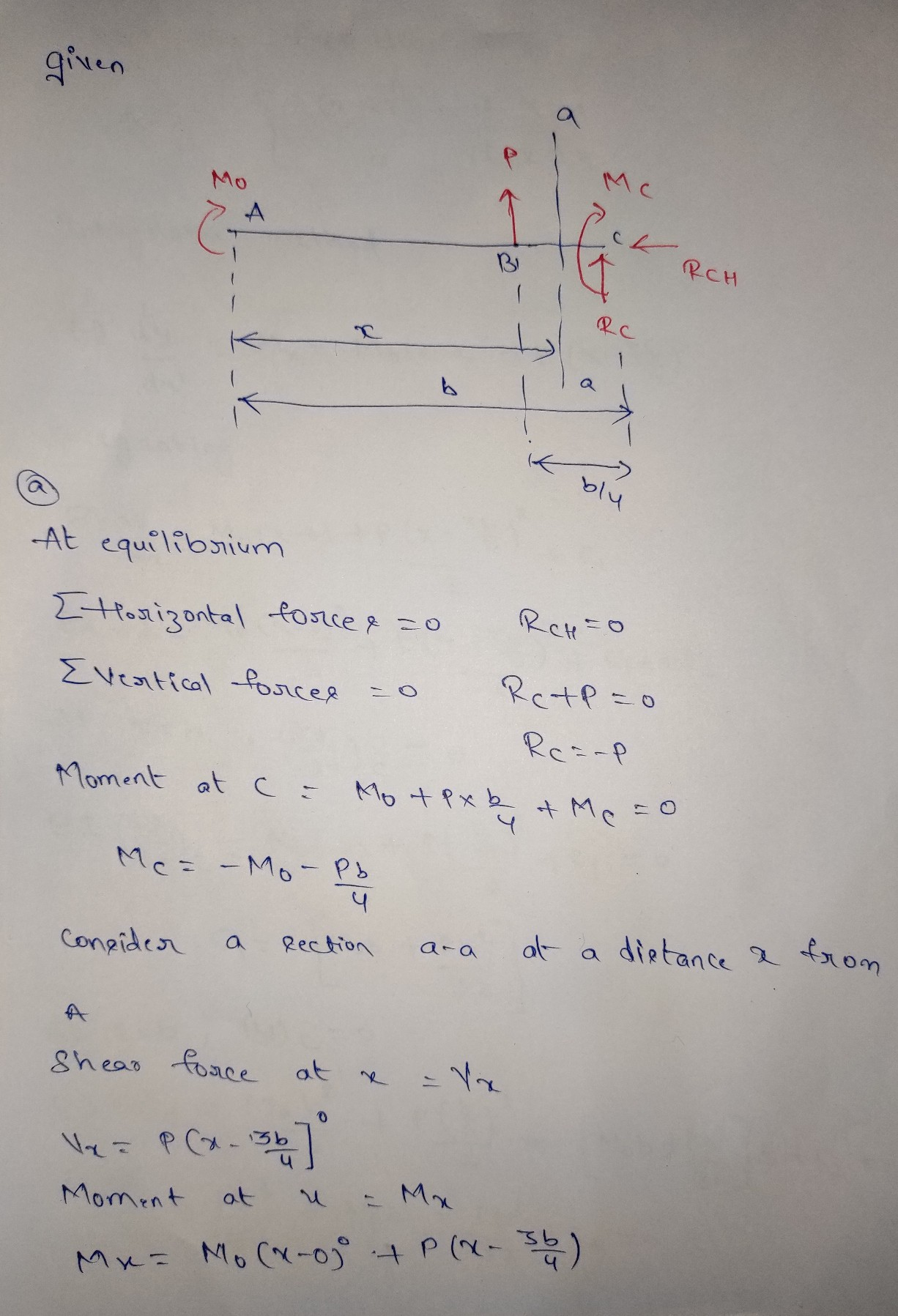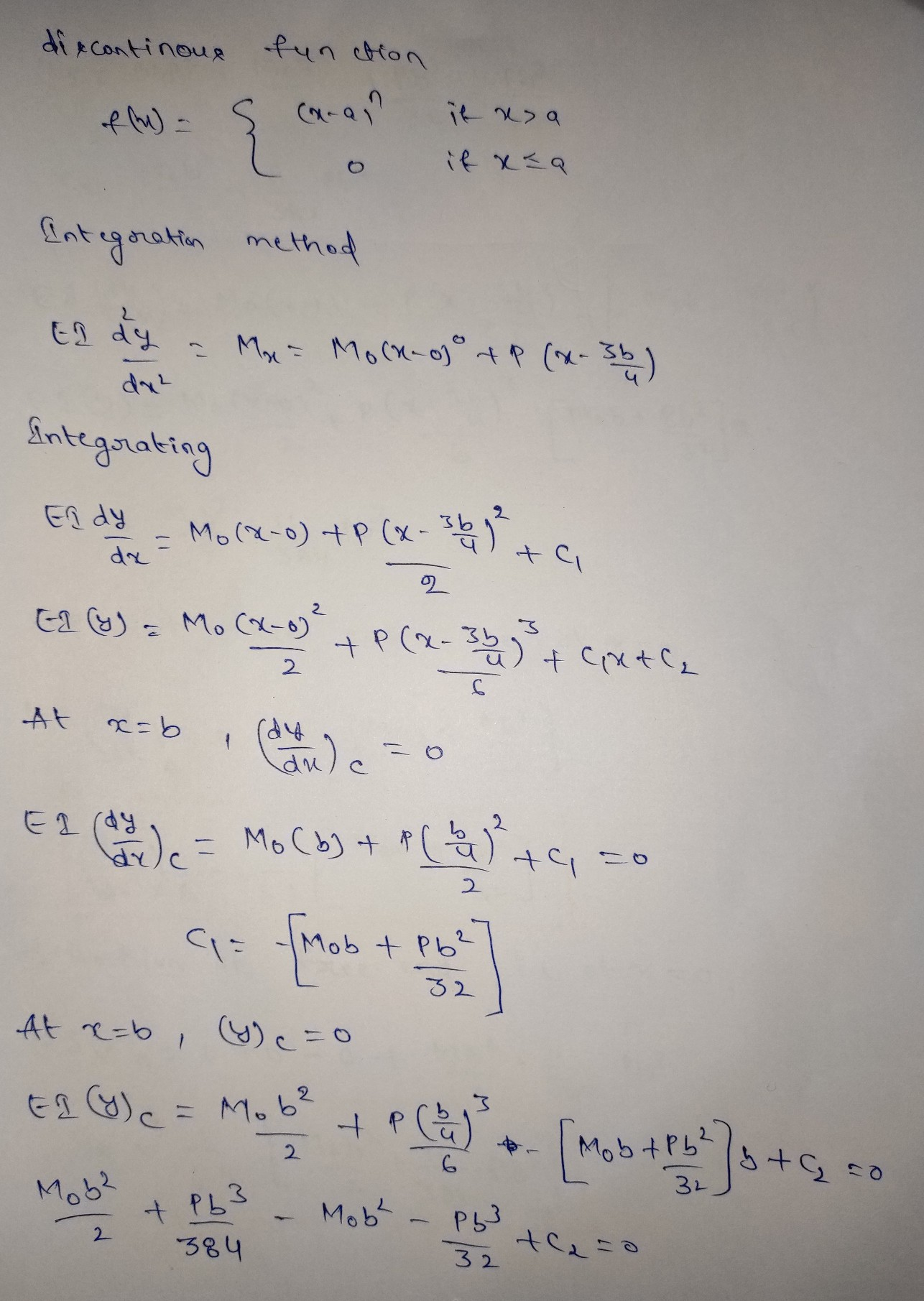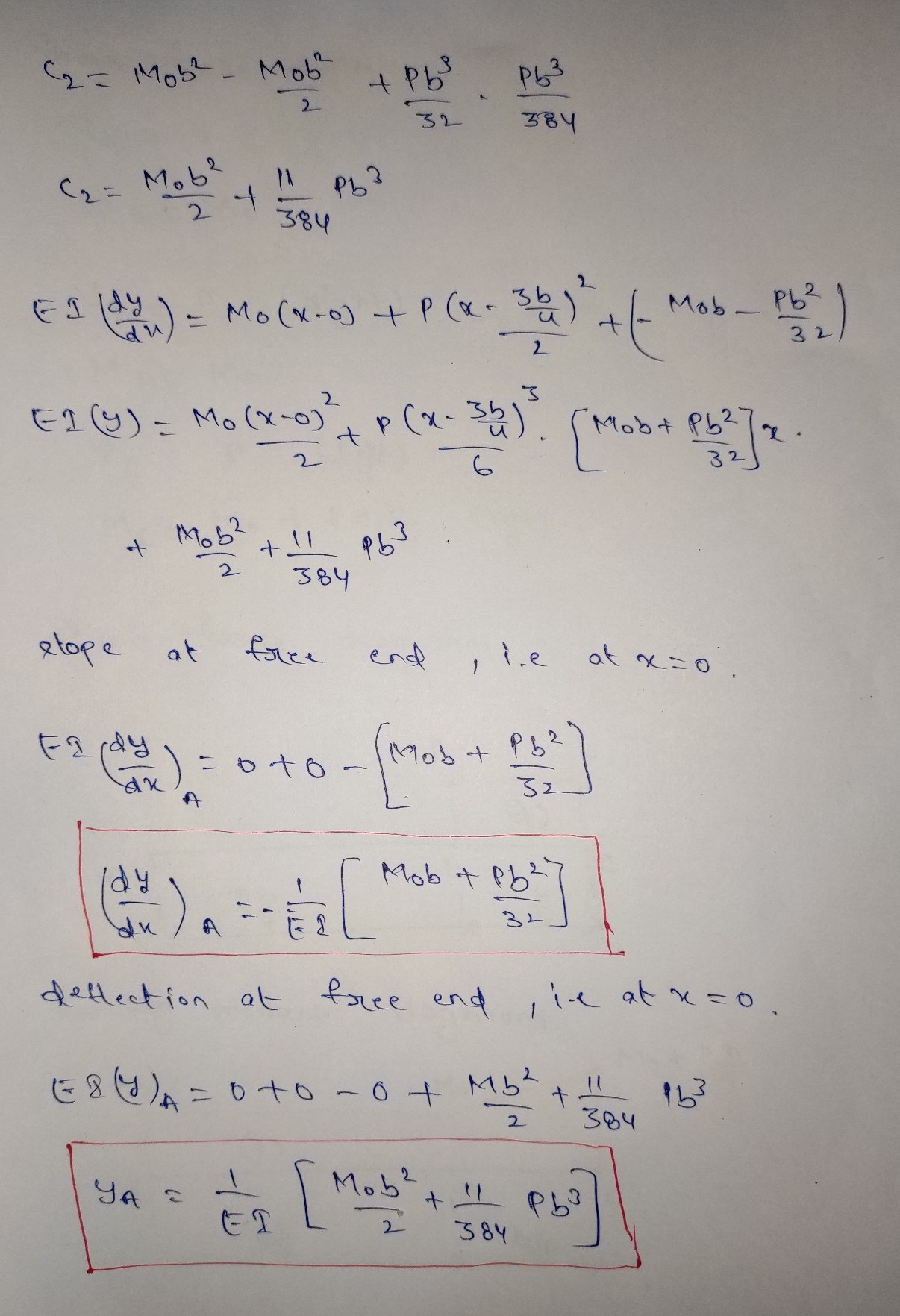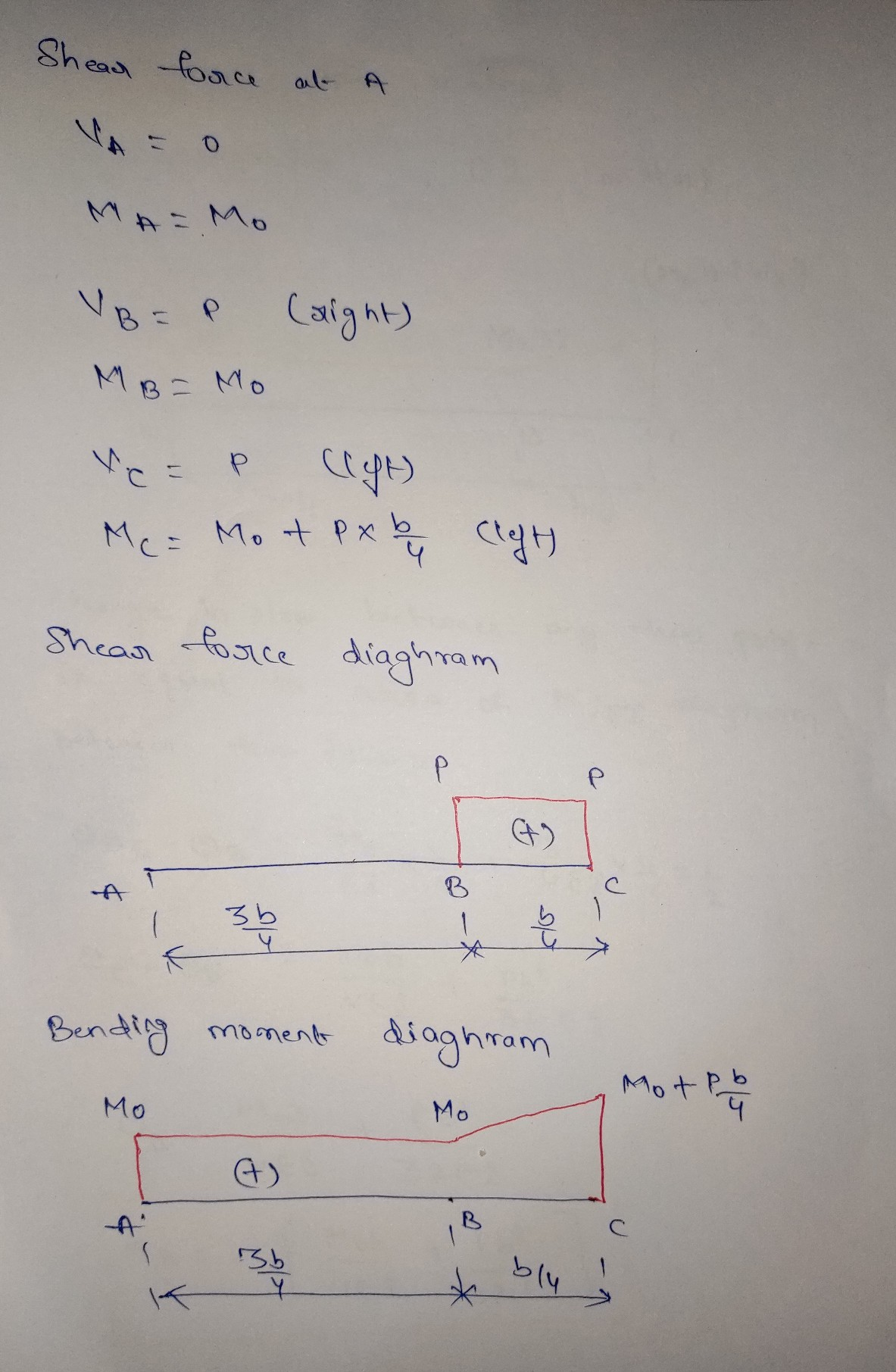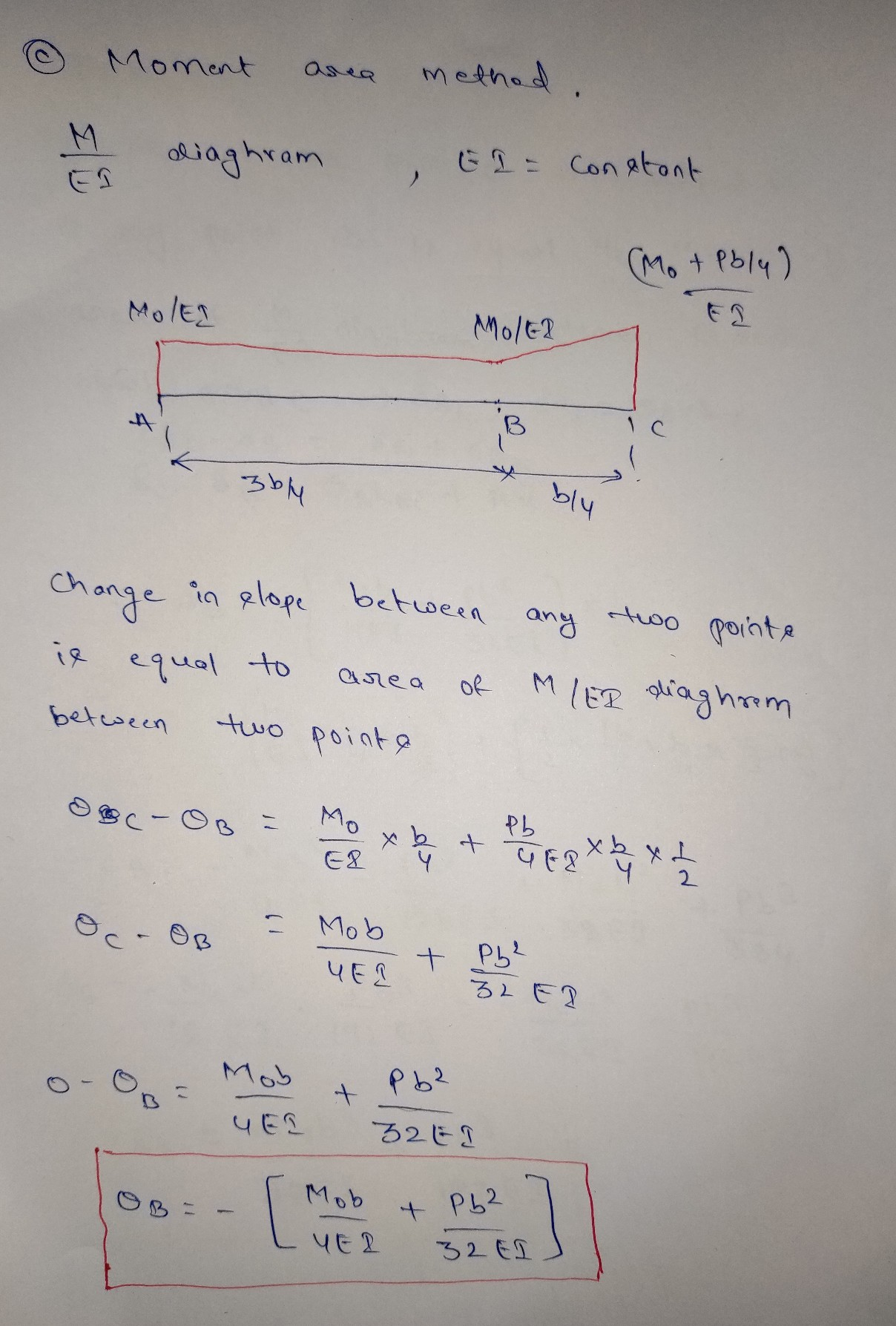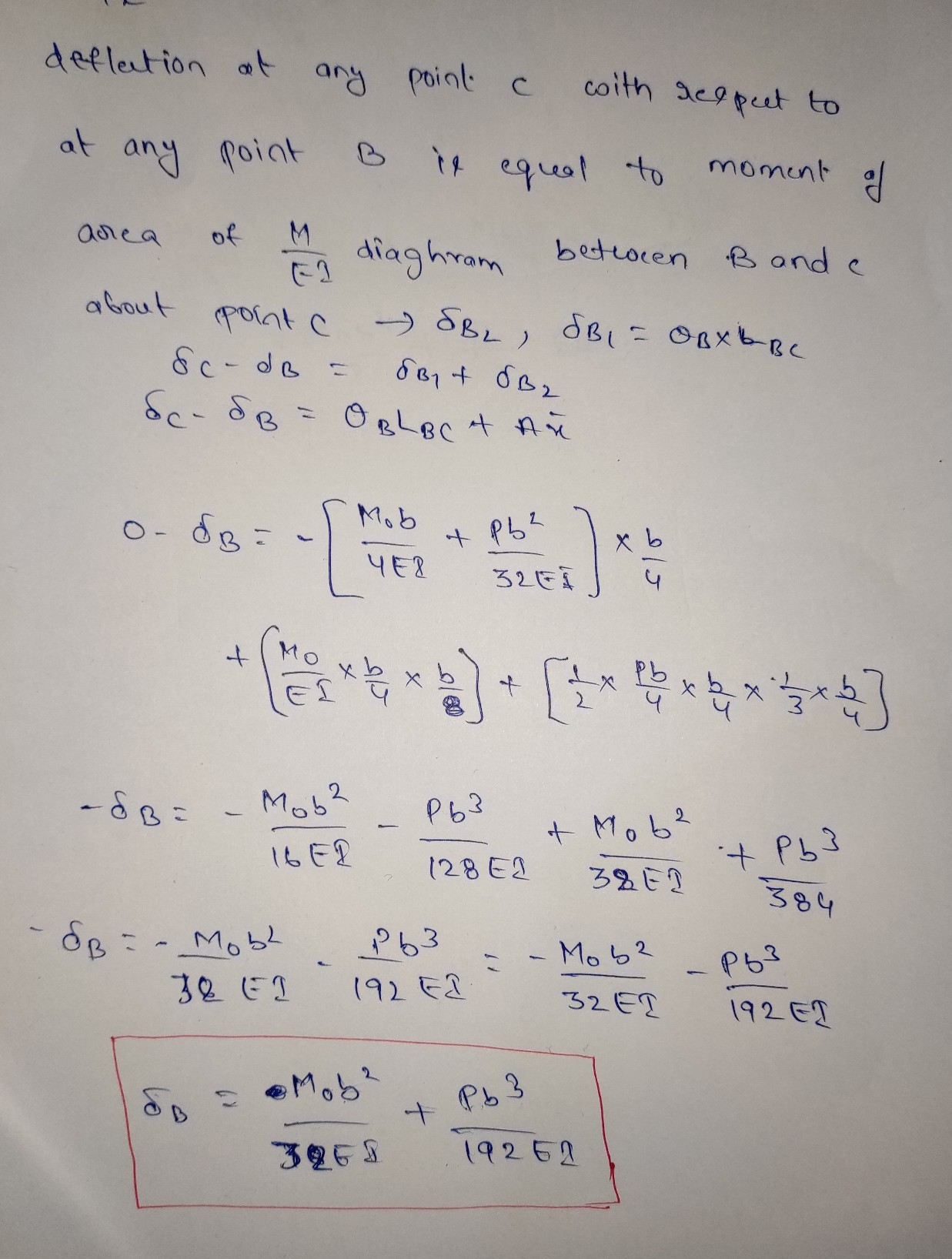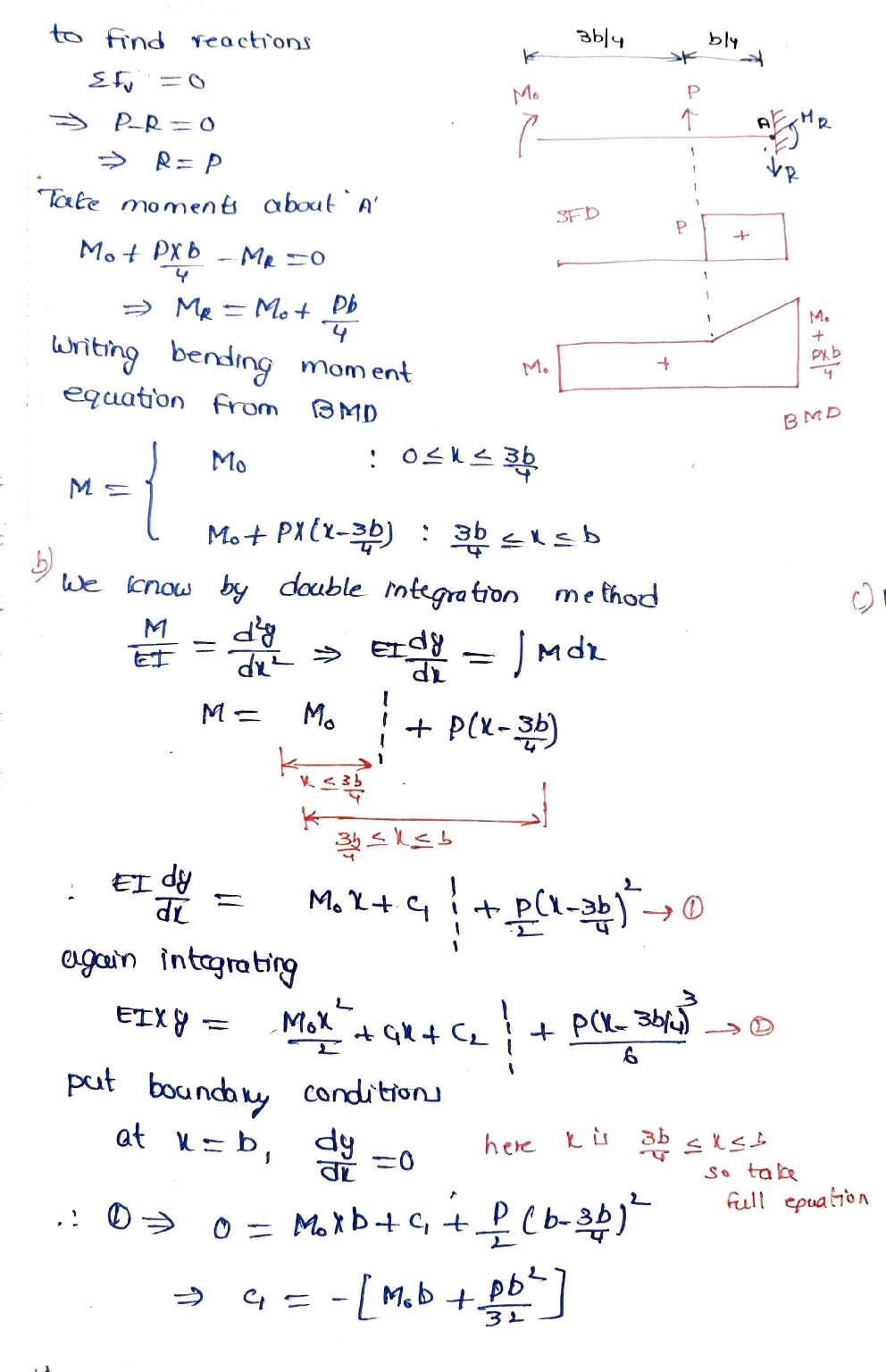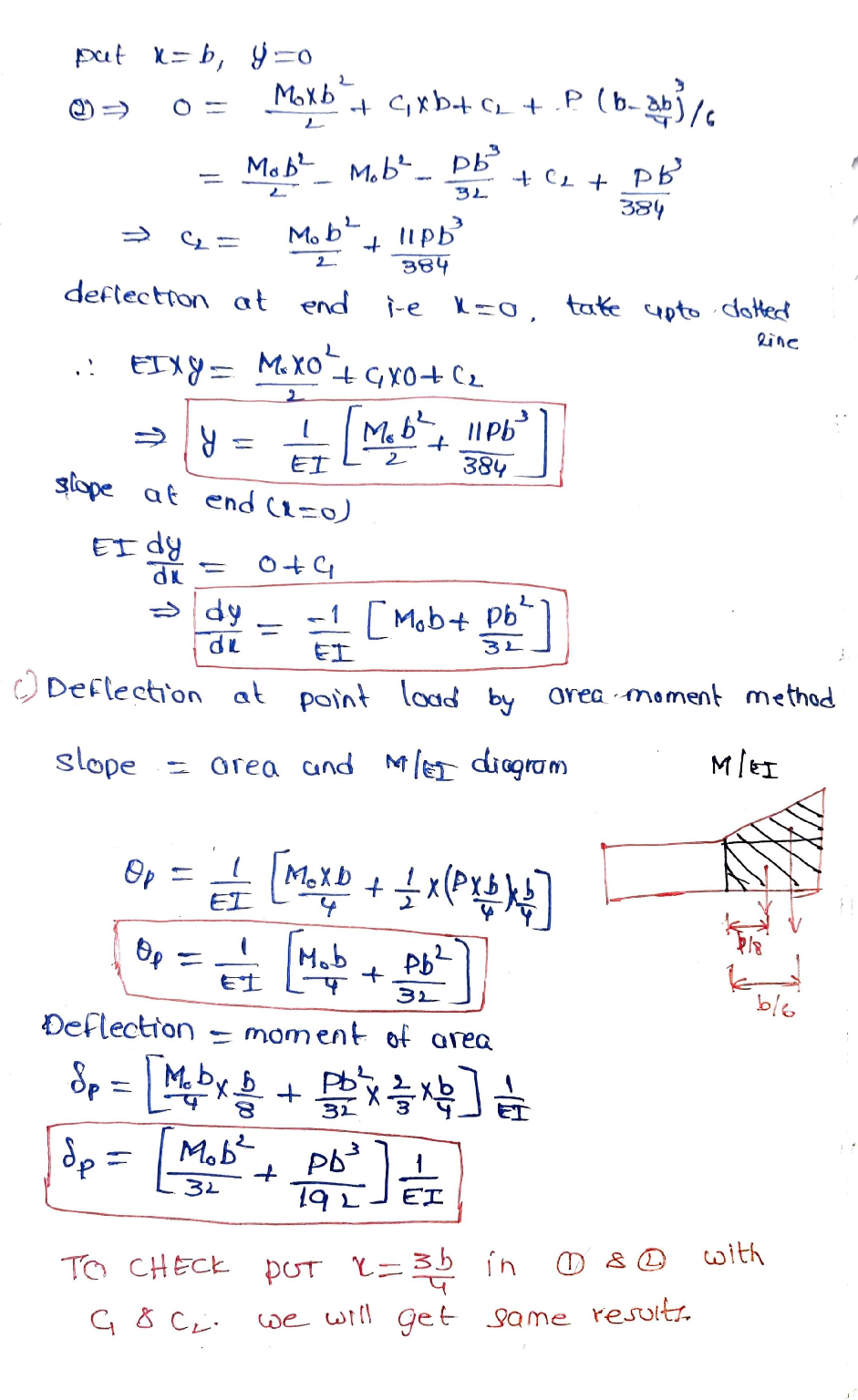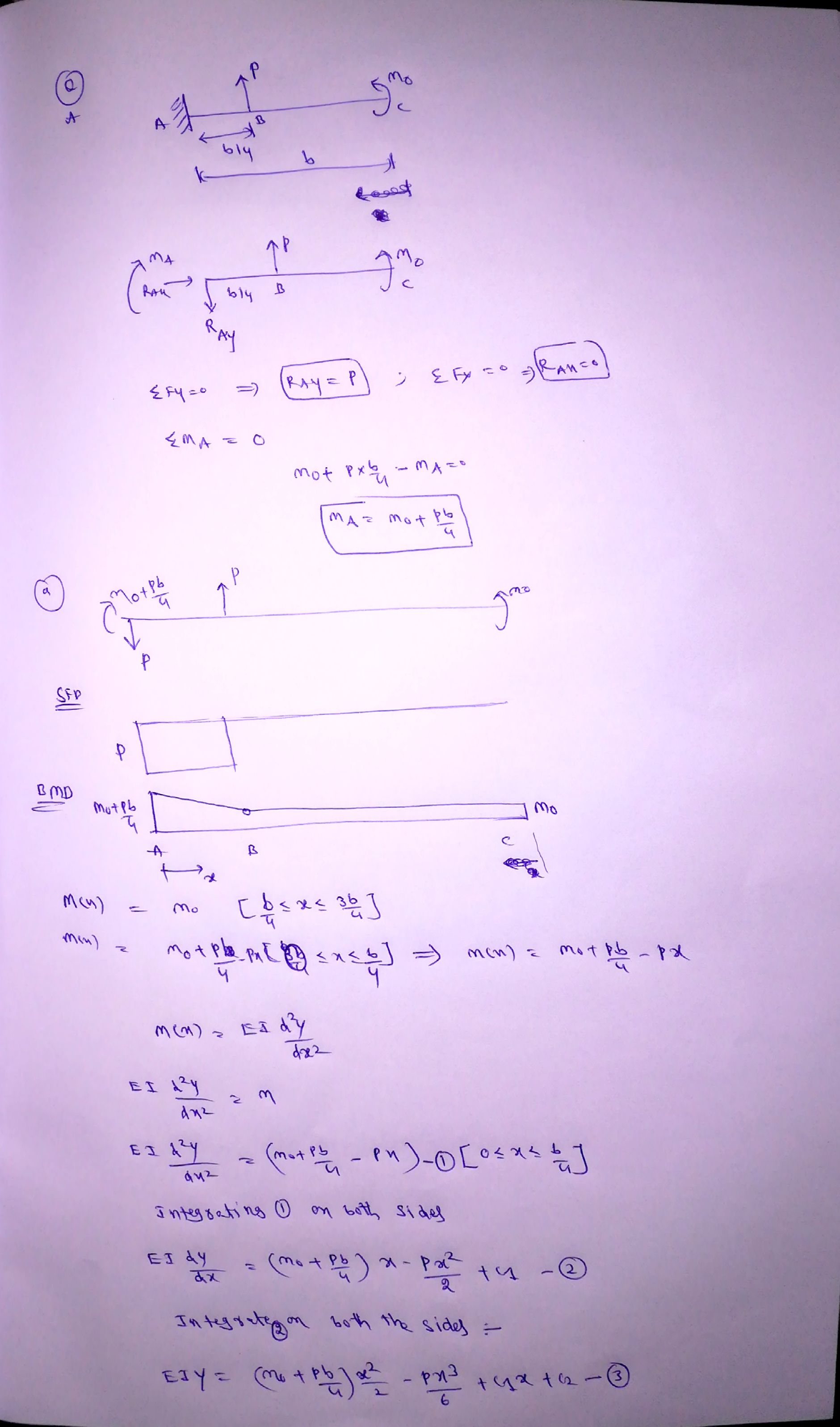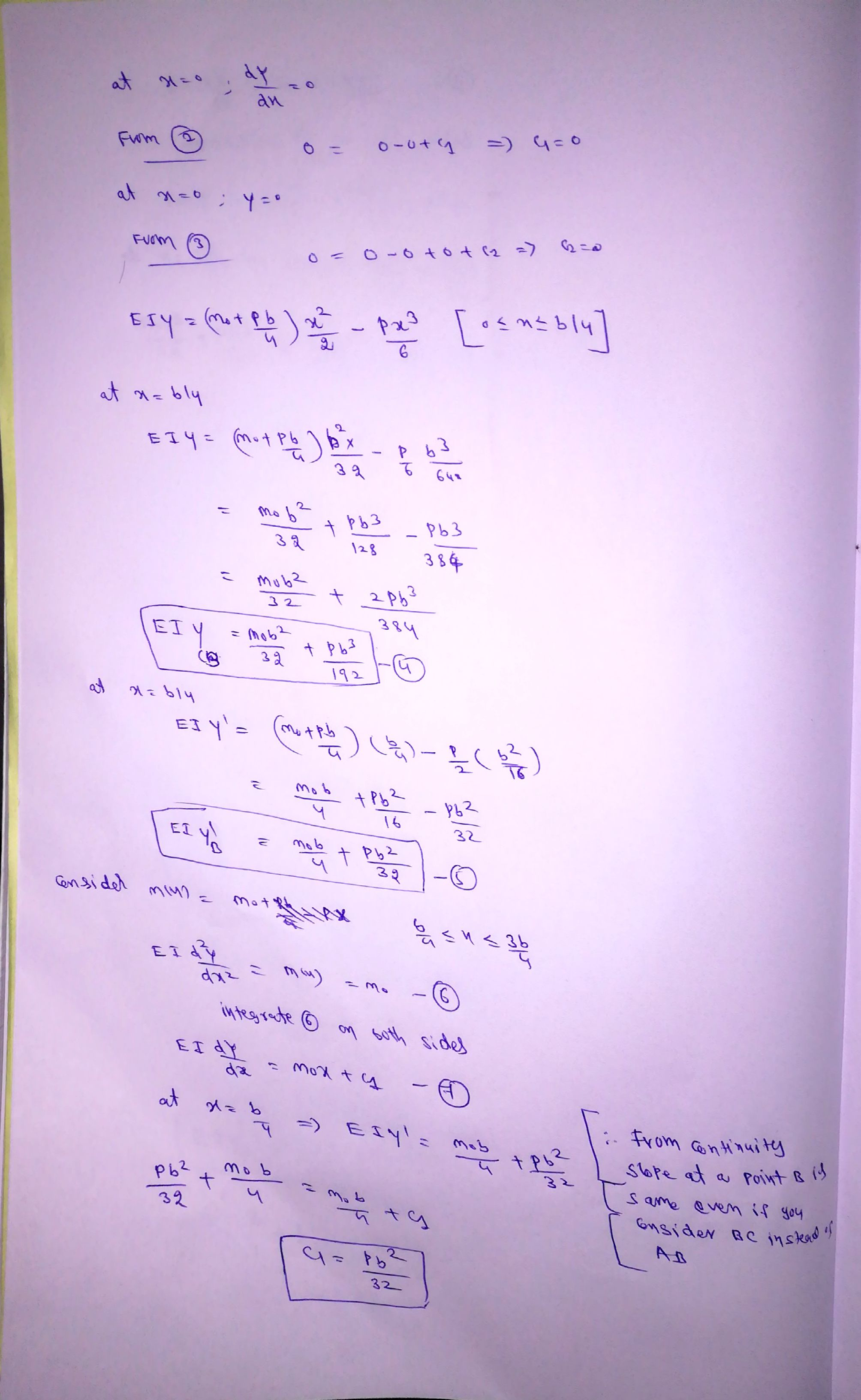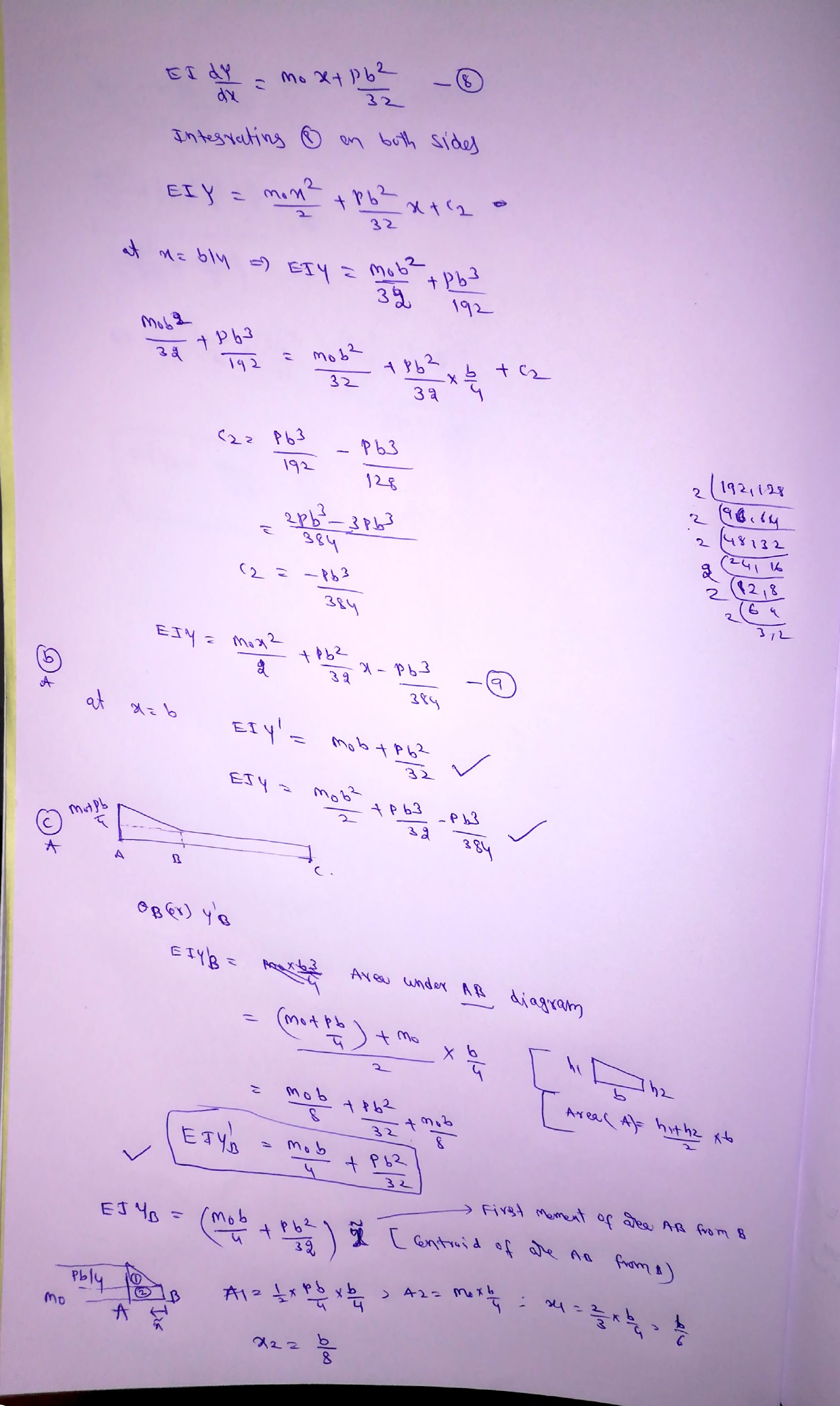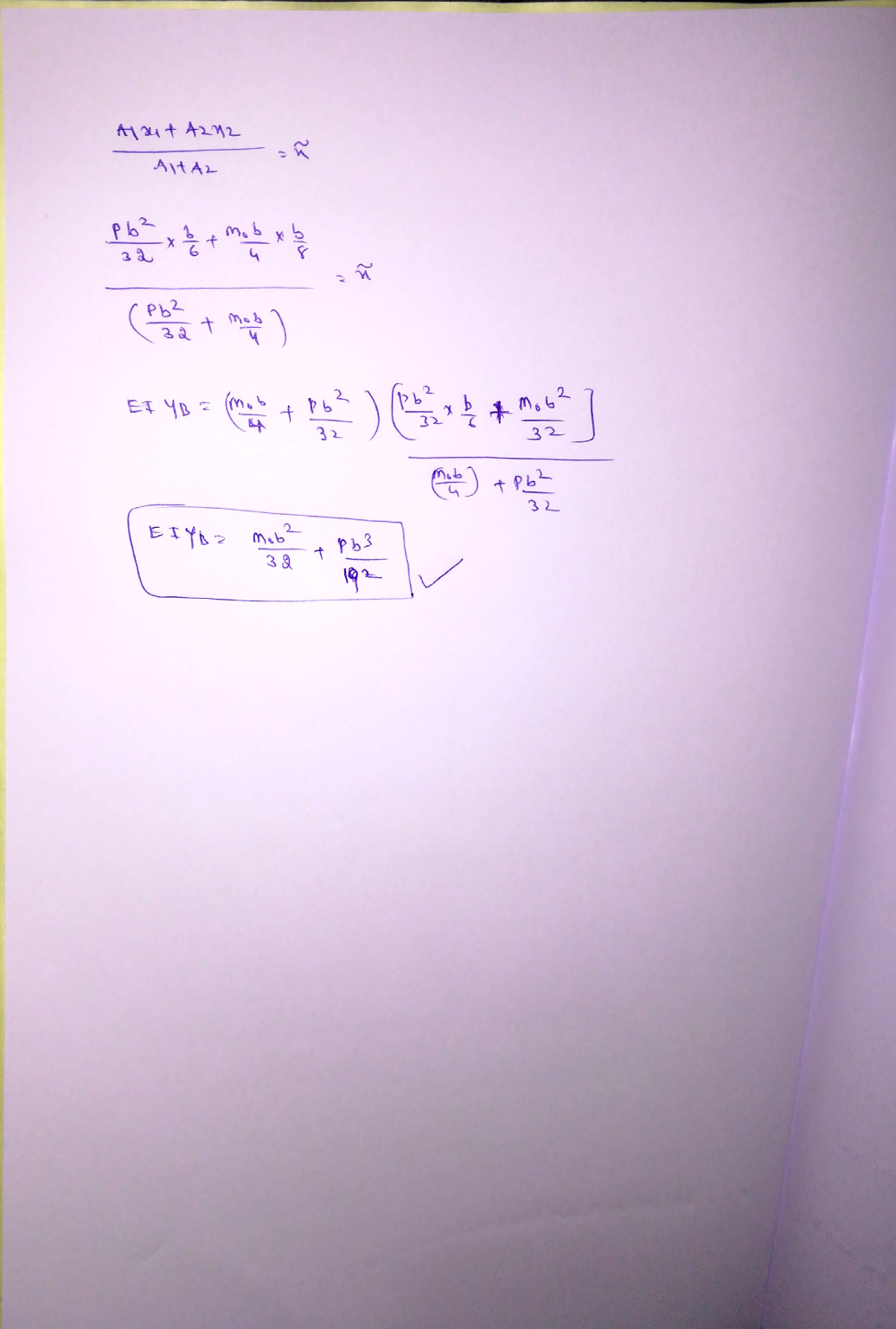#### Earn Coins

Coins can be redeemed for fabulous gifts.

Similar Homework Help Questions
• ### 2. A beam with a uniform flexural rigidity, EI, is loaded by a triangular distributed load,...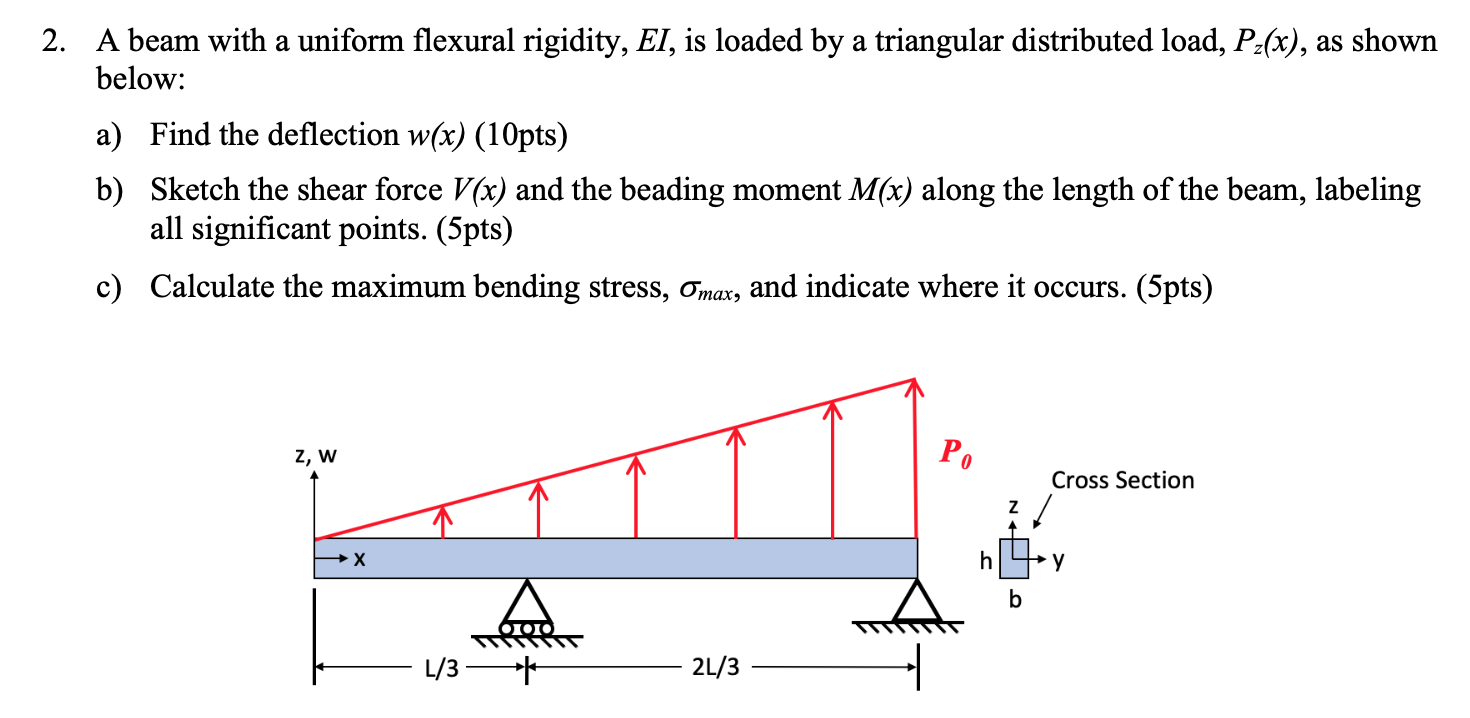2. A beam with a uniform flexural rigidity, EI, is loaded by a triangular distributed load, Pz(x), as shown below: a) Find the deflection w(x) (10pts) b) Sketch the shear force V(x) and the beading moment M(x) along the length of the beam, labeling all significant points. (5pts) c) Calculate the maximum bending stress, Omax, and indicate where it occurs. (5pts) z, W Cross Section - 1/3 — * - 2/3 —

• ### PLEASE PROVIDE THE SLOPE AND DEFELCTION DIAGRAM!!!!!!!!!!!!!! 7) A beam is supported and loaded as shown...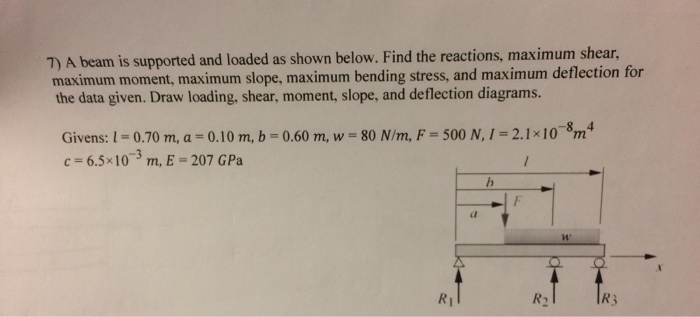PLEASE PROVIDE THE SLOPE AND DEFELCTION DIAGRAM!!!!!!!!!!!!!! 7) A beam is supported and loaded as shown below. Find the reactions, maximum shear, maximum moment, maximum slope, maximum bending stress, and maximum deflection for the data given. Draw loading, shear, moment, slope, and deflection diagrams. -8 4 Givens: 1 0.70 m, a = 0.10 m, b 0.60 m, w 80 N/m, F = 500 N,1 = 2.1x10m c 6.5x10 m, E = 207 GPa / R3 R1 R2 7) A beam...

• ### 7) A beam is supported and loaded as shown below. Find the reactions, maximum shear, maximum...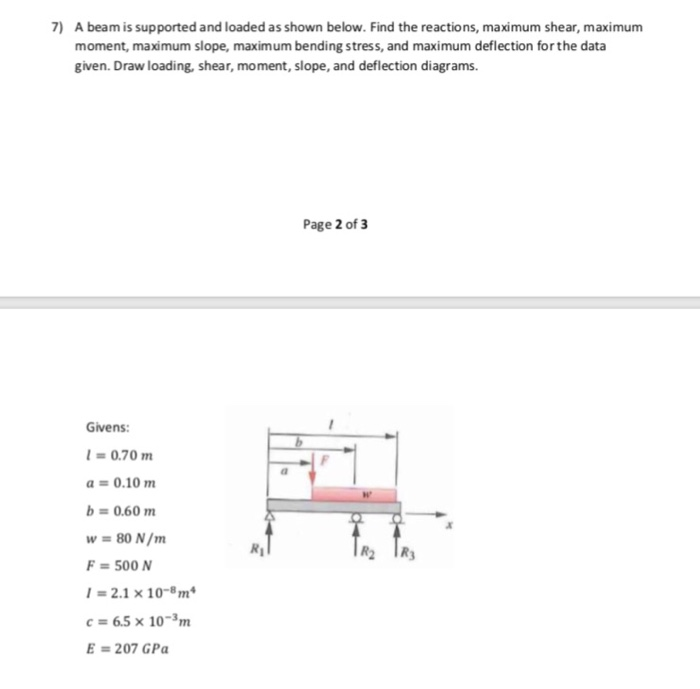7) A beam is supported and loaded as shown below. Find the reactions, maximum shear, maximum moment, maximum slope, maximum bending stress, and maximum deflection for the data given. Draw loading, shear, moment, slope, and deflection diagrams. Page 2 of 3 Givens: 0.70 m a 0.10 m b 0.60 m w 80 N/m F 500 N 2.1 x 10- m c 6.5 x 10-3m E=207 GPa 7) A beam is supported and loaded as shown below. Find the reactions, maximum...

• ### A propped cantilever beam is loaded as shown. Assume that EI = 250,000 kN-m2, and use...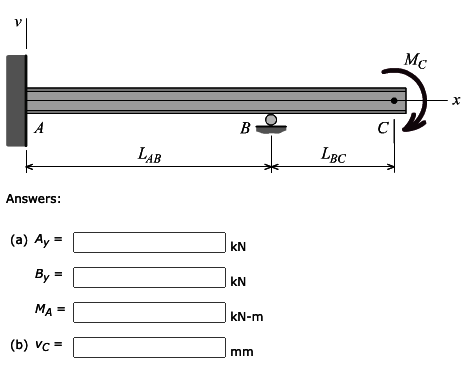A propped cantilever beam is loaded as shown. Assume that EI = 250,000 kN-m2, and use discontinuity functions to determine (a) the reactions at A and B. (b) the beam deflection at C. The reaction forces are positive if up and negative if down. The reaction moment is positive if counterclockwise and negative if clockwise. Assume LAB = 5.4 m, LBC = 2.9 m, MC = 700 kN-m. V Mc X A B LAB LBC Answers: (a) Ay = KN...

• ### For the loading shown in the below figure, knowing that wo 2 kN/m, the length of the beam is L 2 ...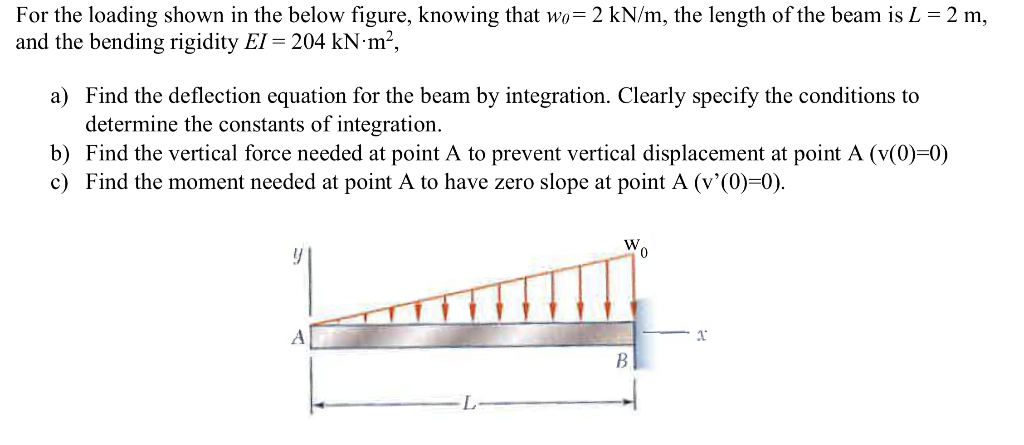For the loading shown in the below figure, knowing that wo 2 kN/m, the length of the beam is L 2 m, and the bending rigidity EI-204 kN-m2, a) Find the deflection equation for the beam by integration. Clearly specify the conditions to determine the constants of integration b) Find the vertical force needed at point A to prevent vertical displacement at point A (v(0)-0) c) Find the moment needed at point A to have zero slope at point A...

• ### Using moment area method, for the beam shown in Figure P-2 find deflection at the center (point C) and rotation under the concentrated load (point D). Also, find locat...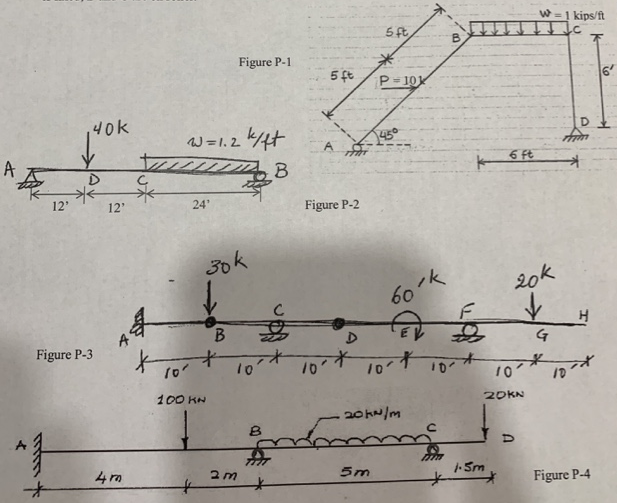2 - Using moment area method, for the beam shown in Figure P-2 find deflection at the center (point C) and rotation under the concentrated load (point D). Also, find location and value of the maximunm deflection. EI constant. 3- Repeat Problem 2 where I for CB is twice as large as I for AC. 4 - For the beam shown in Figure P-3, find the reactions and draw shear and moment diagrams. A is fixed, B and D are hinges, and...

• ### Problem 9.4 The W10x15 cantilevered beam is made of A-36 steel and is subjected to the...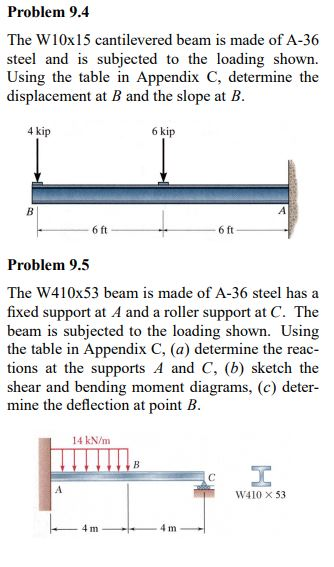Problem 9.4 The W10x15 cantilevered beam is made of A-36 steel and is subjected to the loading shown. Using the table in Appendix C, determine the displacement at B and the slope at B. 4 kip 6 kip В 6 ft 6 ft Problem 9.5 The W410x53 beam is made of A-36 steel has a fixed support at A and a roller support at C. The beam is subjected to the loading shown. Using the table in Appendix C, (a)...

• ### Consider the beam and loading shown. Assume that the flexural rigidity Elof the beam is constant...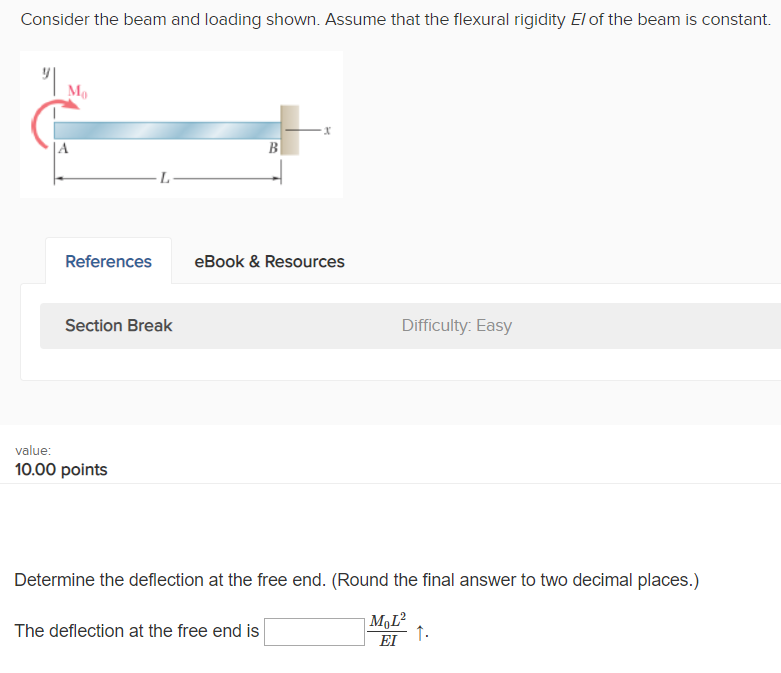Consider the beam and loading shown. Assume that the flexural rigidity Elof the beam is constant Mo References eBook &Resources Section Break Difficulty. Easy value: 10.00 points Determine the deflection at the free end. (Round the final answer to two decimal places.) The deflection at the free end is EI

• ### 4N Problem 6. The beam shown is loaded with a linear distributed load for the left...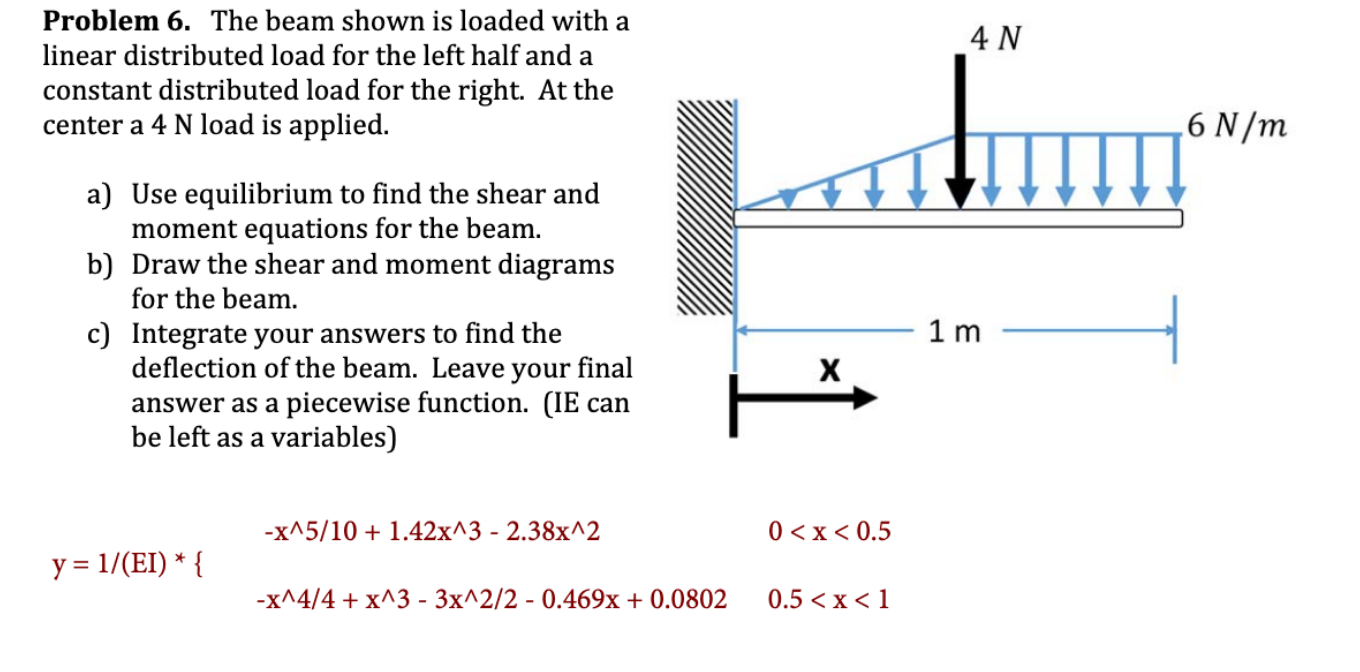4N Problem 6. The beam shown is loaded with a linear distributed load for the left half and a constant distributed load for the right. At the center a 4 N load is applied. 6 N/m a) Use equilibrium to find the shear and moment equations for the beam. b) Draw the shear and moment diagrams for the beam. c) Integrate your answers to find the deflection of the beam. Leave your final answer as a piecewise function. (IE can...

• ### 2. For the beam loaded as shown in the figure, find the following The beam is...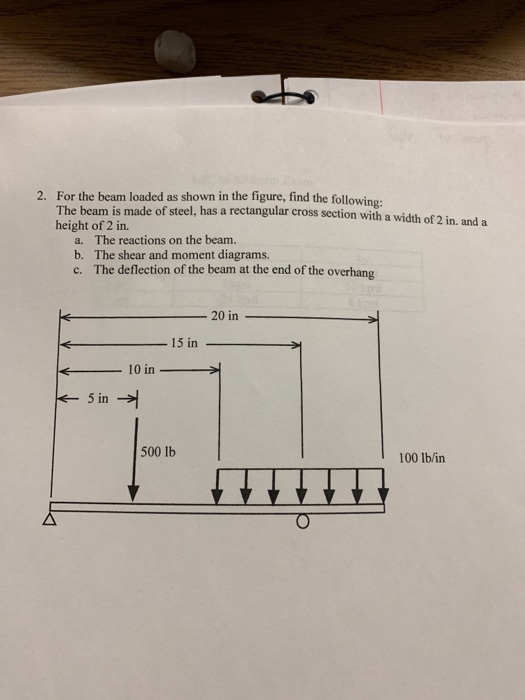2. For the beam loaded as shown in the figure, find the following The beam is made of steel, has a rectangular cross section with a width of 2 in. and a height of 2 in. a. b. c. The reactions on the beam. The shear and moment diagrams. The deflection of the beam at the end of the overhang 20 in 15 in 10 in 500 lb 100 lb/in